# Oranges

Mother divided her three children's oranges in a ratio of 6:5:4. Two children gave 45 oranges. How many oranges were there?

Result

n =  75

#### Solution:Leave us a comment of example and its solution (i.e. if it is still somewhat unclear...):

Showing 0 comments:Be the first to comment!#### To solve this example are needed these knowledge from mathematics:

Need help calculate sum, simplify or multiply fractions? Try our fraction calculator. Do you have a linear equation or system of equations and looking for its solution? Or do you have quadratic equation?

## Next similar examples:

1. New ratio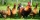The ratio of ducks and chicken in our yard is 2 : 3. The total number of ducks and chickens together is 30. Mother gave 3 of the chickens to our neighbor. What is the new ratio now?
2. Mom and daughterMother is 39 years old. Her daughter is 15 years. For many years will mother be four times older than the daughter?
3. Holidays - on poolChildren's tickets to the swimming pool stands x € for an adult is € 2 more expensive. There was m children in the swimming pool and adults three times less. How many euros make treasurer for pool entry?
4. Three monksThree medieval monks has task to copy 600 pages of the Bible. One rewrites in three days 1 page, second in 2 days 3 pages and a third in 4 days 2 sides. Calculate for how many days and what day the monks will have copied whole Bible when they begin Wednesd
5. PC disksPeter has 45 disks in three colors. One-fifth of the disks are blue, red are twice more than the white. How much is blue, red and white disks?
6. Repair company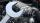The company repairs cars. The first day repair half of the contract second day, the half of the rest and third day 8 residue cars. How many total cars company repaired?
7. DivisorsThe sum of all divisors unknown odd number is 2112. Determine sum of all divisors of number which is twice of unknown numbers.
8. The painterIn order for the painter to get the desired color, he must mix green and yellow in a ratio of 4: 7. If it has 28 l of green color, how many liters of yellow color should he add? How many liters of mixed color does he get?
9. Supermarket 2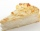A supermarket had a buko pie sale. In the morning 2/3 of the pies were sold and in the afternoon 1/6 of the pies were sold. If 150 pies were left, how many pies had been sold? Show your solution.
10. Soaps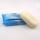Each box has the same number of soaps. A quarter of all boxes contain only white soaps, and in each of the remaining 120 boxes there are always half the white soaps and half the green. White soaps total 1200. (a) the number of all soap boxes; (b) the sma
11. Mushrooms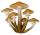For five days, we have collected 410 mushrooms. Interestingly every day we have collected 10 mushrooms more than the preceding day. How many mushrooms we have collected during 4th day?
12. Walnutsx walnuts were in the mission. Dano took 1/4 of nuts Michael took 1/8 from the rest and John took 34 nuts. It stayed here 29 nuts. Determine the original number of nuts.
13. Find the 7Find the number that is smaller than 5 5/12 by as much as 2 2/13 is smaller than 6 1/6
14. Simple equation 6Solve equation with one variable: X/2+X/3+X/4=X+4
15. Fifth of the numberThe fifth of the number is by 24 less than that number. What is the number?
16. Unknown number 23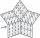Find 2/3 of unknown number, which is two thirds of the 99.
17. On a farm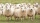On a farm, there are 90 rabbits, 700 sheep, 300 cattle and 500 pigs. What percentage of the total number of animals are rabbits?